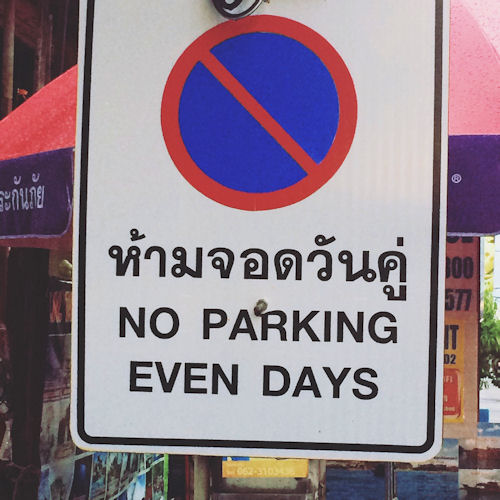# Mix and Math

##### Level 1Level 2Level 3ChallengeDescriptionMore Number

Prime × Prime =

Prime × Composite =

Composite × Prime =

Composite × Composite =

Have you ever noticed that when you add an even number to another even number the sum is always even? Above you can see many similar descriptions of calculations. Click on the link which describes the correct result.

Check

Well done.

You have completed this level and can claim a trophy.

## Description

Determine the nature of adding, subtracting and multiplying numbers with specific properties.

CloseLevel 1 - Even and Odd Numbers

Level 2 - Positive and Negative Numbers

Level 3 - Prime and Composite Numbers

Challenge - Can you find numbers that will satisfy the Mix And Math Challenge?

Answers to this exercise are available lower down this page when you are logged in to your Transum account. If you don’t yet have a Transum subscription one can be very quickly set up if you are a teacher, tutor or parent.

## Definitions

Even numbers are exactly divisible by two.

Odd numbers are not exactly divisible by two.

Positive numbers are greater than zero.

Negative numbers are less than zero.

Prime numbers only have two factors.

Composite numbers have more than two factors.

The solutions to this and other Transum puzzles, exercises and activities are available here when you are signed in to your Transum subscription account. If you do not yet have an account and you are a teacher, tutor or parent you can apply for one by completing the form on the Sign Up page.

Saturday, June 3, 2017

Transum,

Monday, January 8, 2018

"Magic trick:

Ask a friend to hold an odd number of coins in one hand and an even number of coins in the other but not to let you see. You will be able to work out in which hand the odd number of coins is hidden.

Ask the friend to multiply the number of coins in their left hand by three and the number of coins in their right hand by four then add the two totals together.

If the sum of their totals is odd then the odd number of coins is in their left hand otherwise the odd number of coins is in their right hand.

Can you figure out how this works?

For more mathematical magic tricks see Mathemagic."

Do you have any comments? It is always useful to receive feedback and helps make this free resource even more useful for those learning Mathematics anywhere in the world. Click here to enter your comments.

## ExtensionOn how many days of this year is parking allowed?

The solutions to this and other Transum puzzles, exercises and activities are available when you are signed in to your Transum subscription account. If you do not yet have an account and you are a teacher, tutor or parent you can apply for one by completing the form on the Sign Up page.